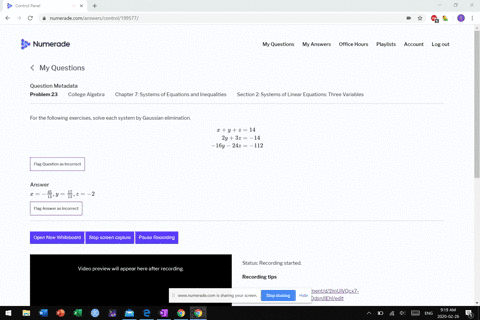Enroll in one of our FREE online STEM summer camps. Space is limited so join now!View Summer Courses### For the following exercises, solve each system by…

03:15
MB

Need more help? Fill out this quick form to get professional live tutoring.

Get live tutoring
Problem 22

For the following exercises, solve each system by Gaussian elimination.
\begin{aligned} 10 x+2 y-14 z &=8 \\-x-2 y-4 z &=-1 \\-12 x-6 y+6 z &=-12 \end{aligned}

$x=2, y=0, z=0$

## Discussion

You must be signed in to discuss.

## Video Transcript

all right. Another galaxy and elimination problem. Um, so I'm gonna live through these and figure out what variable toe make the same and all the equations. Um, I think I'm going with the wise Simon and multiply this equation. Times three, huh? To make it be six. Why wth this equation by negative three to may this be six. Why the situation? I think one and so that made that b six. Why? Also? So the result of doing that would be, um, 30 x three, x plus six. Why? Minus 42 z equals 24. Then times negative three means I get through yet. Plus, it's why, um and then plus 12 equals three. Then then finally, 12 x plus. It's why, um Meyer, 60 meatballs, right? So then I must attract these two equations. Distracted. I'm gonna subtract also trying down here. Okay, So 30 minus three is 27. It's and then negative 42 minus 12. It's negative, or Z, and that equals 24 months. Three is 21. Then the same thing with these I must attract these. I'll subtract them up. Bottom minus the top. So 12 minus three is nine next and then six months. 60 Negative. Six months. 12 on a team. See Equals, um 12 minus three. It's not. Yeah. And then, uh, multiply the bottom equation by free will make the exes be the same. Right? So I get 27. Yes, minus 54 Z equals 27. And then again, I could subtract these two equations and I get zero equals six as false. So what we have here is a case. So you have parallel planes. The beads will have no solution. You comment? Yeah. So there's no unique solution.